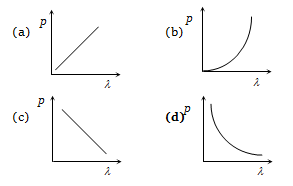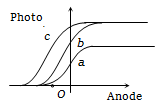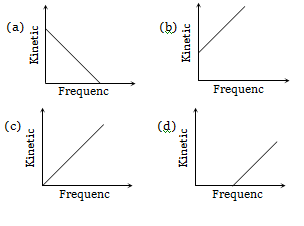The maximum velocity of an electron emitted by light of wavelength $\mathrm{\lambda }$ incident on the surface of a metal of work function $\mathrm{\varphi }$ is
(a) ${\left[\frac{2\left(\mathrm{hc}+\mathrm{\lambda \varphi }\right)}{\mathrm{m\lambda }}\right]}^{1/2}$        (b) ${\left[\frac{2\left(\mathrm{hc}+\mathrm{\lambda \varphi }\right)}{\mathrm{m\lambda }}\right]}^{1/2}$
(c) ${\left[\frac{2\left(\mathrm{hc}-\mathrm{\lambda \varphi }\right)}{\mathrm{m\lambda }}\right]}^{1/2}$        (d) ${\left[\frac{2\left(\mathrm{h\lambda }-\mathrm{\varphi }\right)}{\mathrm{m}}\right]}^{1/2}$

Where h = Planck's constant, m = mass of electron and c = speed of light.

Concept Questions :-

Einstein photo-electric equation
High Yielding Test Series + Question Bank - NEET 2020

Difficulty Level:

In a photoemissive cell with executing wavelength $\mathrm{\lambda }$, the fastest electron has speed v. If the exciting wavelength is changed to 3$\mathrm{\lambda }$/4, the speed of the fastest emitted electron will be
(a) $\mathrm{v}{\left(3/4\right)}^{1/2}$                              (b) $\mathrm{v}{\left(4/3\right)}^{1/2}$
(c) Less than $\mathrm{v}{\left(4/3\right)}^{1/2}$               (d) Greater than $\mathrm{v}{\left(4/3\right)}^{1/2}$

Concept Questions :-

Photoelectric effect experiment
High Yielding Test Series + Question Bank - NEET 2020

Difficulty Level:

Photoelectric emission is observed from a metallic surface for frequencies ${\mathrm{v}}_{1}$ and ${\mathrm{v}}_{2}$ of the incident light rays $\left({\mathrm{v}}_{1}>{\mathrm{v}}_{2}\right)$. If the maximum values of kinetic energy of the photoelectrons emitted in the two cases are in the ratio of 1:k, then the threshold frequency of the metallic surface is

(a) $\frac{{\mathrm{v}}_{1}-{\mathrm{v}}_{2}}{\mathrm{k}-1}$           (b) $\frac{{\mathrm{kv}}_{1}-{\mathrm{v}}_{2}}{\mathrm{k}-1}$
(c) $\frac{{\mathrm{kv}}_{2}-{\mathrm{v}}_{1}}{\mathrm{k}-1}$         (d) $\frac{{\mathrm{v}}_{2}-{\mathrm{v}}_{1}}{\mathrm{k}}$

Concept Questions :-

Electron emission
High Yielding Test Series + Question Bank - NEET 2020

Difficulty Level:

The ratio of de-Broglie wavelengths of molecules of hydrogen and helium which are at temperature 27 $°\mathrm{C}$ and 127 $°\mathrm{C}$ respectively is
(a) $\frac{1}{2}$

(b) $\sqrt{\frac{3}{8}}$
(c) $\sqrt{\frac{8}{3}}$

(d) 1

Concept Questions :-

De-broglie wavelength
High Yielding Test Series + Question Bank - NEET 2020

Difficulty Level:

A photon of wavelength 6630 Å is incident on a totally reflecting surface. The momentum delivered by the photon is equal to

(a) $6.63×{10}^{-27}$ kg-m/sec

(b) $2×{10}^{-27}$ kg-m/sec

(c) ${10}^{-27}$ kg-m/sec

(d) None of these

Concept Questions :-

De-broglie wavelength
High Yielding Test Series + Question Bank - NEET 2020

Difficulty Level:

The ratio of de-Broglie wavelength of a $\mathrm{\alpha }$-particle to that of a proton being subjected to the same magnetic field so that the radii of their path are equal to each other assuming the field induction vector $\stackrel{\to }{\mathrm{B}}$ is perpendicular to the velocity vectors of the $\mathrm{\alpha }$-particle and the proton is
(a) 1

(b) $\frac{1}{4}$
(c) $\frac{1}{2}$

(d) 2

Concept Questions :-

De-broglie wavelength
High Yielding Test Series + Question Bank - NEET 2020

Difficulty Level:

In a photocell bichromatic light of wavelength 2475 Å and 6000 Å are incident on cathode whose work function is 4.8 eV. If a uniform magnetic field of $3×{10}^{-5}$ Tesla exists parallel to the plate, the radius of the path described by the photoelectron will be (mass of electron = $9×{10}^{-31}$ kg)

(a) 1 cm

(b) 5 cm

(c) 10 cm

(d) 25 cm

Concept Questions :-

Einstein photo-electric equation
High Yielding Test Series + Question Bank - NEET 2020

Difficulty Level:

Which of the following figure represents the variation of particle momentum and the associated de-Broglie wavelengthConcept Questions :-

De-broglie wavelength
High Yielding Test Series + Question Bank - NEET 2020

Difficulty Level:

The figure shows the variation of photocurrent with anode potential for a photo-sensitive surface for three different radiations. Let ${\mathrm{I}}_{\mathrm{a}},{\mathrm{I}}_{\mathrm{b}}$ and ${\mathrm{I}}_{\mathrm{c}}$ be the intensities and ${\mathrm{f}}_{\mathrm{a}},{\mathrm{f}}_{\mathrm{b}}$ and ${\mathrm{f}}_{\mathrm{c}}$ be the frequencies for the curves a, b and c respectively(a)
(b)
(c)
(d)

Concept Questions :-

Electron emission
High Yielding Test Series + Question Bank - NEET 2020

Difficulty Level:

According to Einstein's photoelectric equation, the graph between the kinetic energy of photoelectrons ejected and the frequency of incident radiation isConcept Questions :-

Einstein photo-electric equation